# Week 9 Lecture 2: Nonlineardynamics, Bifurcations, and Chaos

## Rahman notes:

### Chaos

While we can't run the numerics on a chaotic system indefinitely, we can for some reasonable amount of time. For the Lorenz equation, we can do this until about t = 35.
y0 = [1; 0; 20];
sigma = 10;
beta = 8/3;
rho = 28;
params = [sigma, beta, rho];
[T,Y] = ode45(@(t, y) Lorenzeq(t, y, params),[0 50],y0);
x1 = Y(:,1); y1 = Y(:,2); z1 = Y(:,3);
t1 = T;
y0 = [1+1e-15; 0; 20];
[T,Y] = ode45(@(t, y) Lorenzeq(t, y, params),[0 50],y0);
x2 = Y(:,1); y2 = Y(:,2); z2 = Y(:,3);
t2 = T;
plot(t1, x1, t2, x2)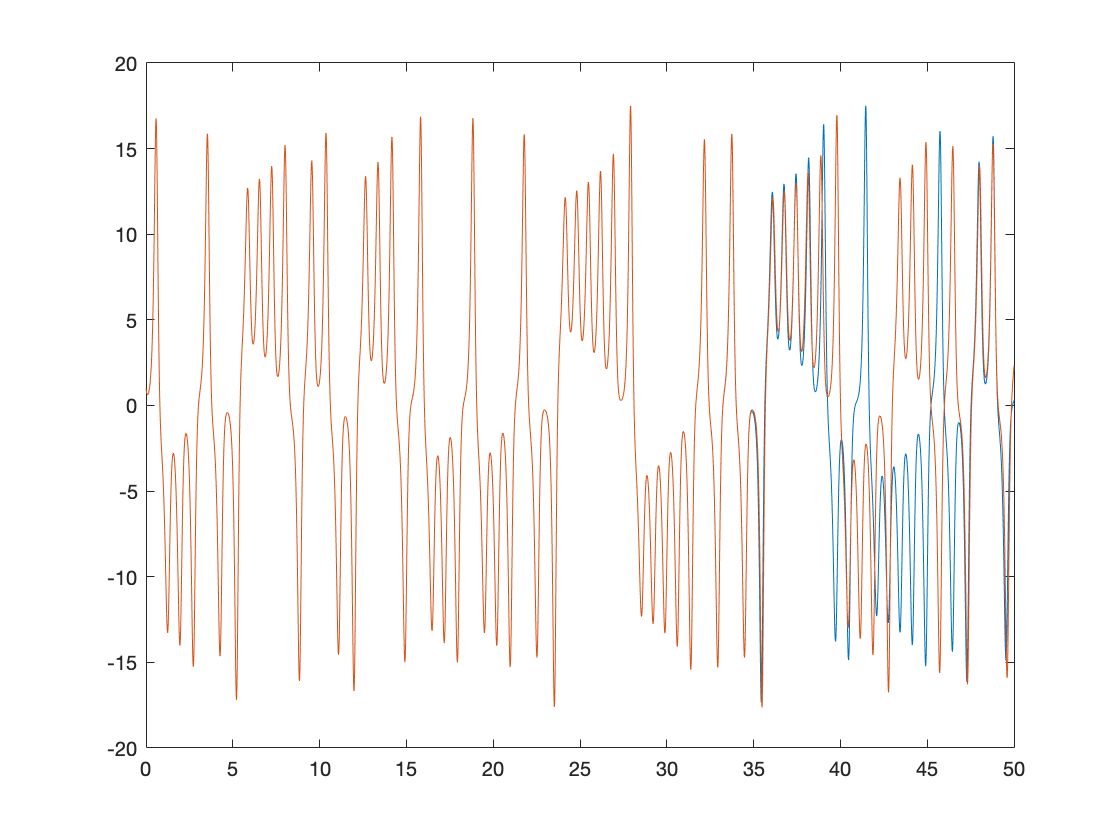After time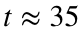, the two solutions are completely different.
Unfortunately, most important physical systems are chaotic. It might sound like this is a hopeless situation - It seems that we can't trust approximations to most interesting physical systems. However, the situation is not as dire as it might seem. While we can't trust the exact values of x or y or z, we can trust more general features of the solution. In particular, the phase diagram for our approximations really is converging to the correct shape.
plot3(x1, y1, z1, x2, y2, z2)
view([-132.1 -31.6])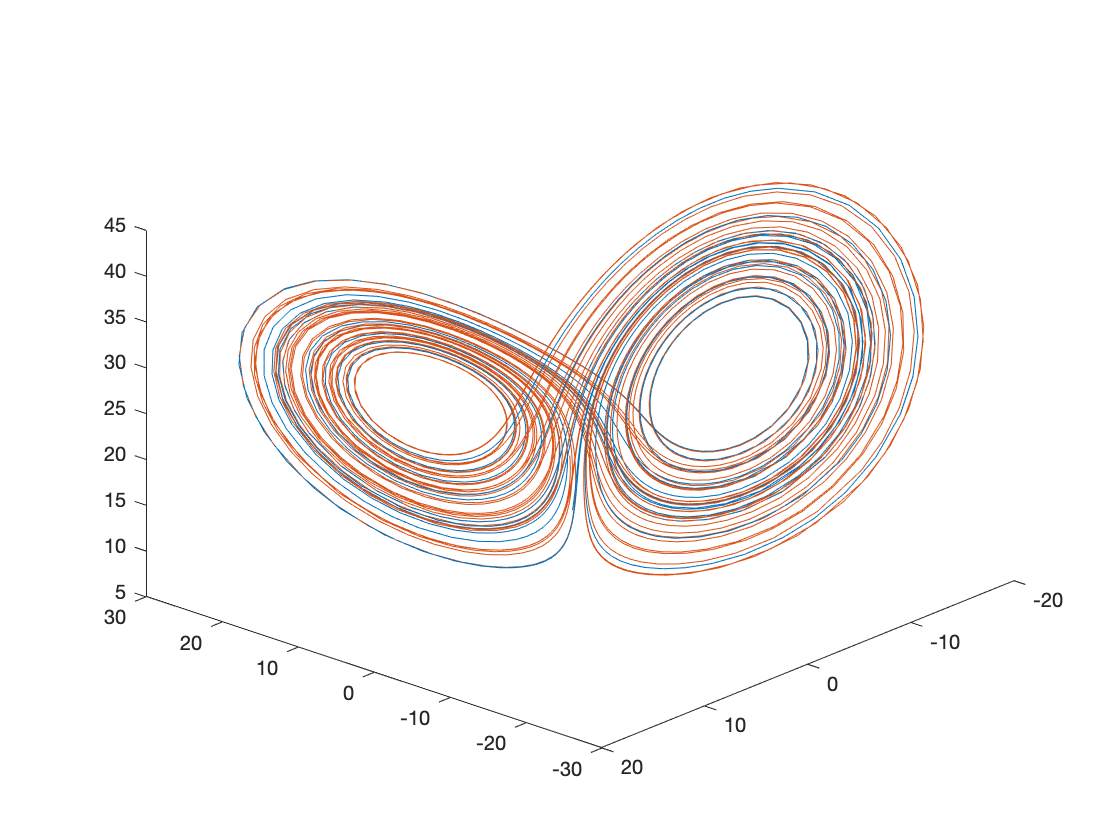This is one of the reasons why we spent so much time worrying about stability. If your differential equation is chaotic, then after some time it doesn't really matter how accurate your solver is. The best you can hope for is to capture the long term stable behavior of the solution. In that case, you care much more about the stability properties of your solver than the accuracy. Remember that implicit methods are generally better at capturing this stable behavior with a larger time step. This means that if you want to know the long-term behavior of a chaotic system, you may be better off using an implicit method like backward Euler or an implicit builtin solver like ode23s instead of a high accuracy explicit solver like RK4.
Now, lets take a step back and see what happnes if we change our parameters.
y0 = [1; 0; 20];
sigma = 10;
beta = 8/3;
count = 1900;
for rho = 28:0.01:30
params = [sigma, beta, rho];
[~,Y] = ode45(@(t, y) Lorenzeq(t, y, params),[0 50],y0);
x = Y(:,1); y = Y(:,2); z = Y(:,3);
count = count + 1;
plot3(x, y, z)
title('Year = ', count)
view([-132.1 -31.6])
xlabel('x')
ylabel('y')
zlabel('z')
axis([-20 20 -30 30 0 70])
pause(0.1)
end
hold on
plot3(x1, y1, z1, '--')
view([-132.1 -31.6])
hold off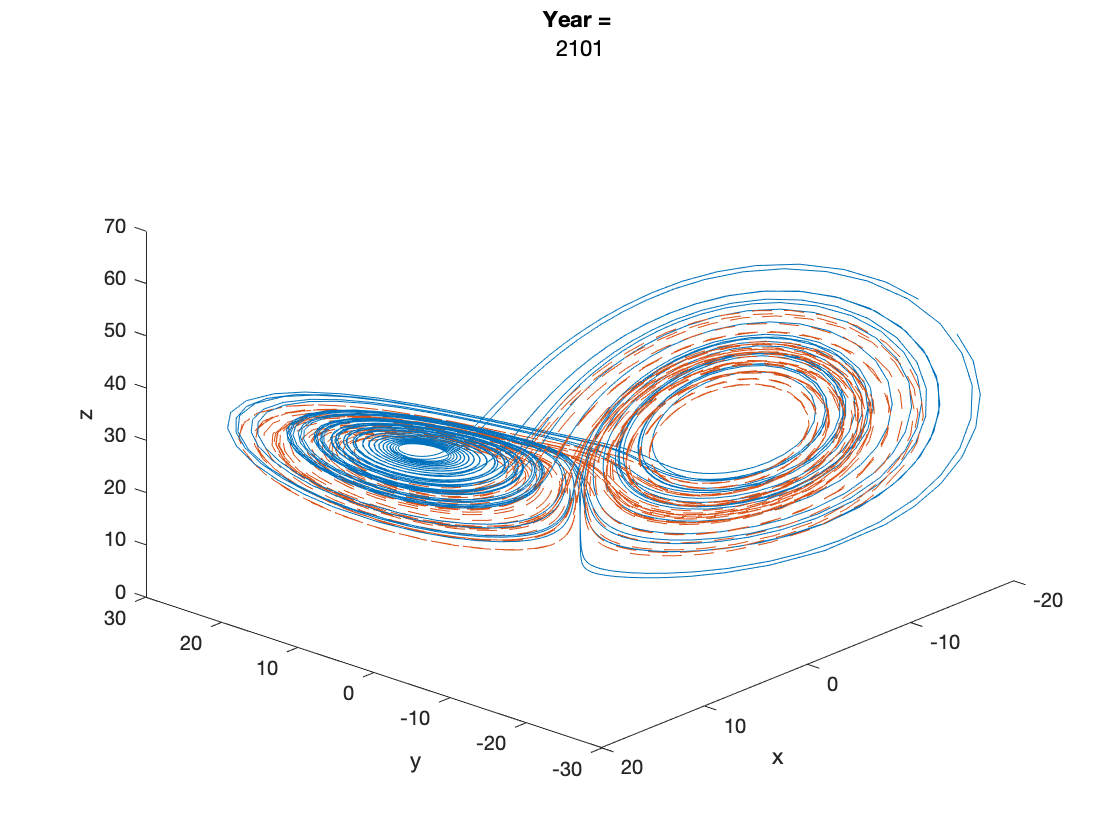### Stiff Differential Equations

There is one other situation where implicit solvers can outperform explicit solvers. To illustrate, let's look at an extremely simplified model for the growth of a spark into a ball of flame. The flame grows because it comes into contact with more oxygen at its surface, and it shrinks because it consumes oxygen in its interior. This means that the rate of growth should be proportional to the surface area of the flame and the rate of decay should be proportional to the volume. This gives us the (highly oversimplified) differential equation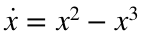.
Here, x is some measure of the size of the flame. SInce the flame starts out as a spark,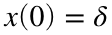should be very small. This model can be solved exactly, and it turns out that the solution stays almost flat at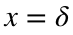for a very long time, then at time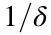it suddenly jumps to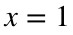. The solution is monotonically increasing, so it should never oscillate up and down. Let's try solving this IVP with ode45. (Remember that ode45 uses a method based on RK4, which is a explicit.)
f = @(t, x)(x^2 - x^3);
delta = 1e-5;
x0 = delta;
tspan = [0 2/delta];
ode45(f, tspan, x0);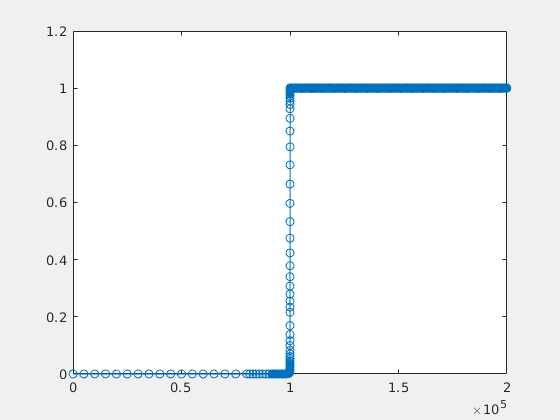Run this code and watch how long it takes to solve, then zoom in on the top half of the graph. You will see that the solution is wildly oscillating up and down instead of staying almost flat as it is supposed to.
The problem is that this differential equation is stiff. There is not a particularly good standard definition of stiffness in differential equations, but the key idea is that the solution operates on two (or more) very different time scales. In our example, the solution changes very slowly most of the time, but there is a moment in the middle when it changes very suddenly. It turns out that explicit solvers have a very difficult time with stiff differential equations. They require a very small time step, and oscillations like this are very common. However, implicit solvers are typically very good at solving stiff problems. For example, we could use the implicit builtin solver ode23s.
We get
ode23s(f, tspan, x0);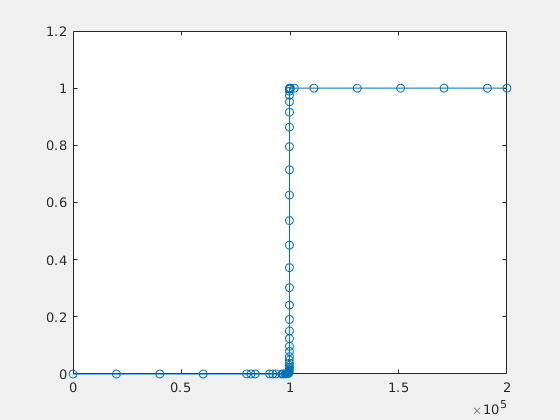Notice that this solver ran much faster with a much bigger time step and did a much better job of capturing the correct behavior.
function dx= Lorenzeq(t, x, params)
sigma = params(1);
beta = params(2);
rho = params(3);
dx1=-sigma*x(1)+sigma*x(2);
dx2=rho*x(1)-x(2)-x(1)*x(3);
dx3=-beta*x(3)+x(1)*x(2);
dx=[dx1;dx2;dx3];
end